(Go Back)
Modeling the Firestik Antenna
by Dick Reid,  KK4OBI

The Firestik line of antennas have been around since 1975… so has the Numerical Electromagnetics Code or NEC program for antenna modeling. One would expect that antenna models for the Firestik type of antenna would be available but nothing can be found on the internet or by asking the Firestik Antenna Company.

Modeling a quarter-wave ground plane antenna like this has some difficulties because the computer versions up to NEC 2 could not accommodate elements touching ground. One related problem is the Automatic Gain Test (AGT) which is used to validate a model. This requires a ground. For the AGT test the “MiniNEC ground” is used to work around this problem. It does this by creating a quarter-wave ground plane near ground to balance the other half of the radiation. For this article the MiniNEC approach was used to simulate a Firestik. A model was constructed with a ground plane of 24 radials set 1 inch over ground. On this radial field was set a 3/8 inch vertical element varying between 2, 3 and 4 feet tall.

How best to load the vertical was a study. Only knowing that the loading section was about 1 foot long, the question was: “How long is the actual loading coil”. To explore this question models were set up using different segment lengths, 4.0 inch and 4.8 inch. This makes it possible for the 4NEC2 antenna modeling program to compare loads of 8.0, 9.6 and 12 inches long positioned on the top of 2, 3 and 4 foot Firestik antennas.

The study was at 27.2 MHz, which is the center of the 40 channel Citzens Band, (CB).

• To resonate the vertical element in the 4NEC2 program do this: Source/Load tab, Load(s), Type, then select Series-RLC.
• Set Symbol R in the range of 10 to 13 Ohms resistance. Not critical.
• Symbol C is essentially stray capacitance in the winding of 5 or 6 pF (10-12 Farad).
• The inductance (Symbol L) is critical... around 16-17 uH (10-6 Henry) for a 2 segment load; 11-12 uH for a 3 segment load.

The optimizer function is used to find the best uH value.

See results of the study in Table 1 following.

 4NEC2 Comparison of Firestik Top Loading Table 1 8 Inch Top Load Gain dBi % Effic. Z Ohms SWR 50 2 Feet -11 40.94 33.0 1.54 3 Feet -7.8 49.58 36.8 1.38 4 Feet -5.8 59.88 39.1 1.28 9.6 Inch Top Load Gain dBi % Effic. Z Ohms SWR 50 2 Feet -11 44.3 28.0 1.63 3 Feet -7.8 50.45 34.7 1.45 4 Feet -5.8 60.44 39.0 1.32 12 Inch Top Load Gain dBi % Effic. Z Ohms SWR 50 2 Feet -12 31.82 37.5 1.33 3 Feet -8.8 40.17 42.5 1.22 4 Feet -6.6 50.51 44.2 1.1

From the above table it is apparent that a 12 inch load produces the lowest % Efficiency.
The 9.6 and 8 inch loading coils have higher % Efficiency.  The 8 inch load offers better SWR. Accordingly, the attached 4NEC2 computer models of 4 Foot, 3 Foot and 2 Foot antennas use 8 inch top loading as the best approximation of a Firestik design.

Mechanical Tuning

As described by KC7VWM, tuning a Firestik antenna is done by a screw that is used to adjust the length of the antenna. To simulate this adjustment method in the 4NEC2 antenna model a programmable symbol “Tune” is incorporated. Using this “Tune” symbol provides the means to predict how much a change in length causes how much change in frequency.

Tuning adjustment study was in steps of 0.05, 0.1 and 0.15 feet above and below the model length at 27.2 MHz. That is equal to steps of 0.6”, 1.2” and 1.8”. At each change in length a Frequency Sweep was made to determine the new Frequency MHz and SWR

The Length change vs. Frequency change results for 4 foot, 3 foot and 2 foot Firestik-type antenna modelss are summarized in the graphs following. Note that all the X and Y axis are identical so all curves are directly comparable.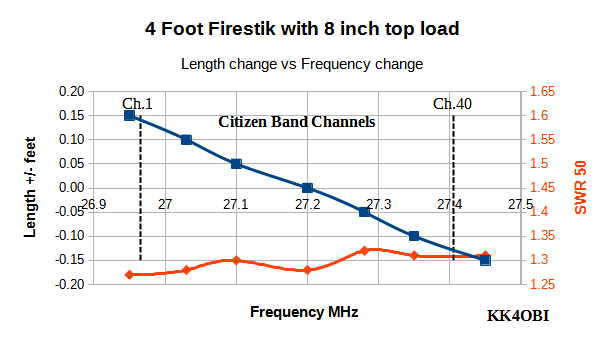Graph 1

In graph 1 the tuning to Channel 1 is predicted to be longer by about 0.14 ft. (1.68”) and Channel 40 about -0.13 ft. (-1.56”) shorter.
The model indicates low SWR with some non-linearity which may be an artifact in modeling.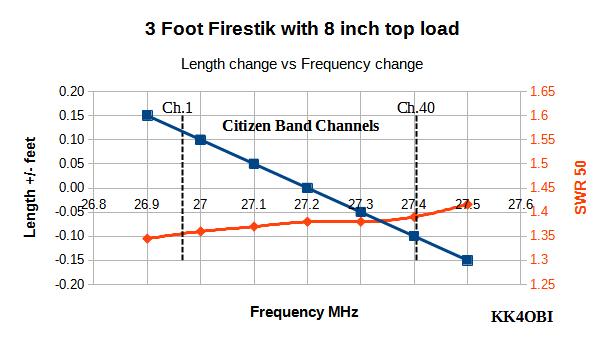Graph 2

In Graph 2 the tuning for Channel 1 is predicted to be longer by about 0.12 ft. (1.44”) and Channel 40 about -0.11 ft. (-1.32”) shorter.
SWR is higher than a 4 Foot Firestik with an almost linear rate of change over the CB band.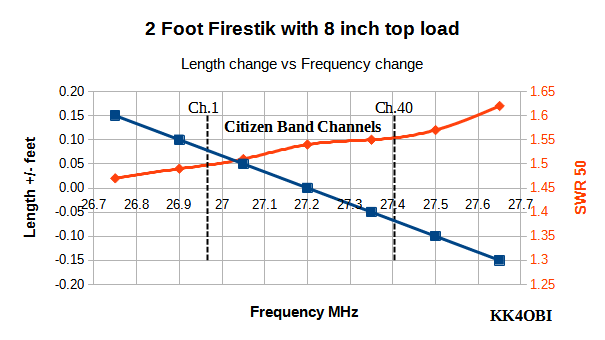Graph 3

Graph 3 shows that tuning is sensitive. Channel 1 tuning is predicted to be only about 0.06 ft. (0.72”) longer and Channel 40 only about -0.07 ft. (-0.84”) shorter.
SWR is higher but still useful. Some slight non-linearity over the CB band is noted.

The radiation pattern (below) is the same for all three antennas. Only the Gain and % Efficiency varies as seen in Table 1..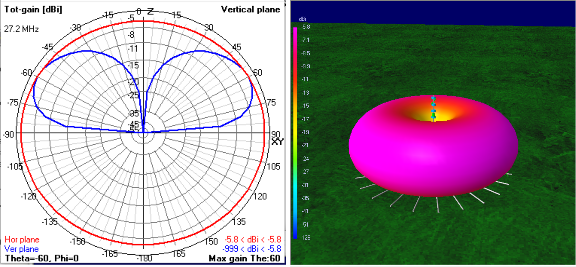Figure 1

Appendix of 4NEC2 model for 4 ft. Firestik antenna

Copy the text between the double horizontal lines and save as Firestik 4ft.NEC.txt

To use the NEC model, remove “.txt” and run Firestik 4ft.NEC

================= Start of 4 foot Firestik antenna model ===========================

CM Since NEC2 can not allow anything touching ground, this model is set to use the "Real" (Sommerfeld) ground so you can make the radials close to ground.. The MiniNEC ground can be used but generally not as good.
CM
CM This is loaded by experinentally finding Inductance, capacitance and resistance on the top two segments of the 4-foot x 3/8"diameter vertical.radiator.
CM 4 FOOT FIRESTIK MODEL
CM Radials are 8 feet of #14 coated wire (.00267radius) with 24 segs. 1 seg=8/20= 4 inches
CM Vertical is 4 feet (48") of 3/8 dia (0.0156 radius) Segs = 48/4== 12. Use 12 segments
CM The model is tuned to mid-band at 27.2 MHz... around channel 20.
CM A "Tune" section is adjusted to any CB channel.Longer = Chan1-20. Shorter = Chan 20-40 (also 10m up to 28.5MHz)
CM The advertised "5/8 wavelength" refers to the length of wire used to wrap the stick... not frequency..
CM
CM
CE
SY hgh=0.083 'In feet 1 inch =0.083 over ground
SY Freq=27.2 'MHz
SY H=1.741e-5 'Henries inductance
SY R=12.92 'Resistance
SY Vert=4 'Height of vertical in feet
SY Tune=0 'Tuning length added to vert
SY Ang1=0 'Angles of each radial
SY Ang2=15
SY Ang3=30
SY Ang4=45
SY Ang5=60
SY Ang6=75
SY Ang7=90 '90 degrees
SY Ang8=105
SY Ang9=120
SY Ang10=135
SY Ang11=150
SY Ang12=165
SY Ang13=180 '180 degrees
SY Ang14=195
SY Ang15=210
SY Ang16=225
SY Ang17=240
SY Ang18=255
SY Ang19=270 '270 degrees
SY Ang20=285
SY Ang21=300
SY Ang22=315
SY Ang23=330
SY Ang24=345
GW 1 12 0 0 hgh 0 0 hgh+Vert+Tune 0.0156 'of Vertical 3/8" radiator with load on top
GW 2 24 0 0 hgh Vert*Sin(ang1) Vert*cos(ang1) hgh Radius 'of #14 coated wire
GW 4 24 0 0 hgh Vert*Sin(ang3) Vert*cos(ang3) hgh Radius
GW 5 24 0 0 hgh Vert*Sin(ang4) Vert*cos(ang4) hgh Radius
GW 6 24 0 0 hgh Vert*Sin(ang5) Vert*cos(ang5) hgh Radius
GW 7 24 0 0 hgh Vert*Sin(ang6) Vert*cos(ang6) hgh Radius
GW 8 24 0 0 hgh Vert*Sin(ang7) Vert*cos(ang7) hgh Radius
GW 9 24 0 0 hgh Vert*Sin(ang8) Vert*cos(ang8) hgh Radius
GW 10 24 0 0 hgh Vert*Sin(ang9) Vert*cos(ang9) hgh Radius
GW 11 24 0 0 hgh Vert*Sin(ang10) Vert*cos(ang10) hgh Radius
GW 12 24 0 0 hgh Vert*Sin(ang11) Vert*cos(ang11) hgh Radius
GW 13 24 0 0 hgh Vert*Sin(ang12) Vert*cos(ang12) hgh Radius
GW 14 24 0 0 hgh Vert*Sin(ang13) Vert*cos(ang13) hgh Radius
GW 15 24 0 0 hgh Vert*Sin(ang14) Vert*cos(ang14) hgh Radius
GW 16 24 0 0 hgh Vert*Sin(ang15) Vert*cos(ang15) hgh Radius
GW 17 24 0 0 hgh Vert*Sin(ang16) Vert*cos(ang16) hgh Radius
GW 18 24 0 0 hgh Vert*Sin(ang17) Vert*cos(ang17) hgh Radius
GW 19 24 0 0 hgh Vert*Sin(ang18) Vert*cos(ang18) hgh Radius
GW 20 24 0 0 hgh Vert*Sin(ang19) Vert*cos(ang19) hgh Radius
GW 21 24 0 0 hgh Vert*Sin(ang20) Vert*cos(ang20) hgh Radius
GW 22 24 0 0 hgh Vert*Sin(ang21) Vert*cos(ang21) hgh Radius
GW 23 24 0 0 hgh Vert*Sin(ang22) Vert*cos(ang22) hgh Radius
GW 24 24 0 0 hgh Vert*Sin(ang23) Vert*cos(ang23) hgh Radius
GW 25 24 0 0 hgh Vert*Sin(ang24) Vert*cos(ang24) hgh Radius
GS 0 0 0.3048
GE 1
LD 0 1 11 12 R H F
LD 5 1 1 12 58000000
LD 7 1 1 12 2.4
GN 2 0 0 0 4 0.003
EK
EX 0 1 1 0 1 0 0
FR 0 0 0 0 Freq 0
EN

============== End of 4 Foot Firestik antenna model ====================================

Appendix of 4NEC2 model for 3 ft. Firestik antenna

Copy the text between the double horizontal lines and save as Firestik 3ft.NEC.txt

To use the NEC model, remove “.txt” and run Firestik 3ft.NEC

====================== Start of 3 Foot Firestik antenna model ============================

CM Since NEC2 can not allow anything touching ground, this model is set to use the "Real" (Sommerfeld) ground so you can make the radials as close to ground.. The MiniNEC ground can be used but generally not as good.
CM
CM This is loaded by experinentally finding Inductance, capacitance and resistance on the top two segments of the 3-foot x 3/8"diameter vertical.radiator.
CM FIRESTIK 3 FT MODEL
CM Radials are 8 feet of #14 coated wire with 24 segs. 1 seg=8/24= 4 inches
CM The model is tuned to mid-band at 27.2 MHz... around channel 20.
CM A "Tune" section is adjusted to any CB channel.Longer = Chan 1-20. Shorter = Chan 20-40
CM The advertised "5/8 wavelength" refers to the length of wire used to wrap the stick... not frequency..
CM
CM
CE
SY hgh=0.083 'In feet 1 inch =0.083 over ground
SY Freq=27.2 'MHz
SY H=1.768e-5 'Henries inductance
SY R=12.92 'Resistance
SY Vert=3 'Height of vertical in feet
SY Tune=0 'Tuning length added to vert
SY Ang1=0 'Angles of each radial
SY Ang2=15
SY Ang3=30
SY Ang4=45
SY Ang5=60
SY Ang6=75
SY Ang7=90 '90 degrees
SY Ang8=105
SY Ang9=120
SY Ang10=135
SY Ang11=150
SY Ang12=165
SY Ang13=180 '180 degrees
SY Ang14=195
SY Ang15=210
SY Ang16=225
SY Ang17=240
SY Ang18=255
SY Ang19=270 '270 degrees
SY Ang20=285
SY Ang21=300
SY Ang22=315
SY Ang23=330
SY Ang24=345
GW 1 9 0 0 hgh 0 0 hgh+Vert+Tune 0.0156 'of Vertical 3/8" radiator with load on top
GW 2 24 0 0 hgh Vert*Sin(ang1) Vert*cos(ang1) hgh Radius 'of #14 coated wire
GW 3 24 0 0 hgh Vert*Sin(ang2) Vert*cos(ang2) hgh Radius
GW 4 24 0 0 hgh Vert*Sin(ang3) Vert*cos(ang3) hgh Radius
GW 5 24 0 0 hgh Vert*Sin(ang4) Vert*cos(ang4) hgh Radius
GW 6 24 0 0 hgh Vert*Sin(ang5) Vert*cos(ang5) hgh Radius
GW 7 24 0 0 hgh Vert*Sin(ang6) Vert*cos(ang6) hgh Radius
GW 8 24 0 0 hgh Vert*Sin(ang7) Vert*cos(ang7) hgh Radius
GW 9 24 0 0 hgh Vert*Sin(ang8) Vert*cos(ang8) hgh Radius
GW 10 24 0 0 hgh Vert*Sin(ang9) Vert*cos(ang9) hgh Radius
GW 11 24 0 0 hgh Vert*Sin(ang10) Vert*cos(ang10) hgh Radius
GW 12 24 0 0 hgh Vert*Sin(ang11) Vert*cos(ang11) hgh Radius
GW 13 24 0 0 hgh Vert*Sin(ang12) Vert*cos(ang12) hgh Radius
GW 14 24 0 0 hgh Vert*Sin(ang13) Vert*cos(ang13) hgh Radius
GW 15 24 0 0 hgh Vert*Sin(ang14) Vert*cos(ang14) hgh Radius
GW 16 24 0 0 hgh Vert*Sin(ang15) Vert*cos(ang15) hgh Radius
GW 17 24 0 0 hgh Vert*Sin(ang16) Vert*cos(ang16) hgh Radius
GW 18 24 0 0 hgh Vert*Sin(ang17) Vert*cos(ang17) hgh Radius
GW 19 24 0 0 hgh Vert*Sin(ang18) Vert*cos(ang18) hgh Radius
GW 20 24 0 0 hgh Vert*Sin(ang19) Vert*cos(ang19) hgh Radius
GW 21 24 0 0 hgh Vert*Sin(ang20) Vert*cos(ang20) hgh Radius
GW 22 24 0 0 hgh Vert*Sin(ang21) Vert*cos(ang21) hgh Radius
GW 23 24 0 0 hgh Vert*Sin(ang22) Vert*cos(ang22) hgh Radius
GW 24 24 0 0 hgh Vert*Sin(ang23) Vert*cos(ang23) hgh Radius
GW 25 24 0 0 hgh Vert*Sin(ang24) Vert*cos(ang24) hgh Radius
GS 0 0 0.3048
GE 1
LD 0 1 8 9 R H F
LD 5 1 1 9 58000000
LD 7 1 1 9 2.4
GN 2 0 0 0 4 0.003
EK
EX 0 1 1 0 1 0 0
FR 0 0 0 0 Freq 0
EN

======================== End of 3 Foot Firestik antenna model.=======================

Appendix of 4NEC2 model for 2 ft. Firestik antenna

Copy the text between the double horizontal lines and save as Firestik 2ft.NEC.txt

To use the NEC model, remove “.txt” and run Firestik 2ft.NEC

========================= Start of 2 Foot Firestik antenna model =========================

CM Since NEC2 can not allow anything touching ground, this model is set to use the "Real" (Sommerfeld) ground so you can make the radials as close to ground.. The MiniNEC ground can be used but generally not as good.
CM
CM This is loaded by experinentally finding Inductance, capacitance and resistance on the top two segments of the 2-foor x 3/8"diameter vertical.radiator.
CM MODEL FIRESTIK 2 FOOT
CM Radials are 8 feet of #14 coated wire with 24 segs. 1 seg=8/24= 4 inches
CM Vertical is 2 feet (24") Segs = 24/4= 6 segments
CM The model is tuned to mid-band at 27.2 MHz... around channel 20.
CM A "Tune" section is adjusted to any CB channel.Longer = Chan1-20. Shorter = Chan 20-40
CM The advertised "5/8 wavelength" refers to the length of wire used to wrap the stick... not frequency..
CM
CM
CE
SY hgh=0.083 'In feet 1 inch =0.083
SY Freq=27.2 'MHz
SY H=1.737e-5 'Henries inductance
SY R=12.92 'Resistance
SY Vert=2 'Height of vertical in feet
SY Tune=0 'Tuning length added to vert
SY Ang1=0 'Angles of each radial
SY Ang2=15
SY Ang3=30
SY Ang4=45
SY Ang5=60
SY Ang6=75
SY Ang7=90 '90 degrees
SY Ang8=105
SY Ang9=120
SY Ang10=135
SY Ang11=150
SY Ang12=165
SY Ang13=180 '180 degrees
SY Ang14=195
SY Ang15=210
SY Ang16=225
SY Ang17=240
SY Ang18=255
SY Ang19=270 '270 degrees
SY Ang20=285
SY Ang21=300
SY Ang22=315
SY Ang23=330
SY Ang24=345
GW 1 6 0 0 hgh 0 0 hgh+Vert+Tune 0.0156 'Vertical radiator with load on top
GW 2 24 0 0 hgh Vert*Sin(ang1) Vert*cos(ang1) hgh Radius '#14 coated wire
GW 3 24 0 0 hgh Vert*Sin(ang2) Vert*cos(ang2) hgh Radius
GW 4 24 0 0 hgh Vert*Sin(ang3) Vert*cos(ang3) hgh Radius
GW 5 24 0 0 hgh Vert*Sin(ang4) Vert*cos(ang4) hgh Radius
GW 6 24 0 0 hgh Vert*Sin(ang5) Vert*cos(ang5) hgh Radius
GW 7 24 0 0 hgh Vert*Sin(ang6) Vert*cos(ang6) hgh Radius
GW 8 24 0 0 hgh Vert*Sin(ang7) Vert*cos(ang7) hgh Radius
GW 9 24 0 0 hgh Vert*Sin(ang8) Vert*cos(ang8) hgh Radius
GW 10 24 0 0 hgh Vert*Sin(ang9) Vert*cos(ang9) hgh Radius
GW 11 24 0 0 hgh Vert*Sin(ang10) Vert*cos(ang10) hgh Radius
GW 12 24 0 0 hgh Vert*Sin(ang11) Vert*cos(ang11) hgh Radius
GW 13 24 0 0 hgh Vert*Sin(ang12) Vert*cos(ang12) hgh Radius
GW 14 24 0 0 hgh Vert*Sin(ang13) Vert*cos(ang13) hgh Radius
GW 15 24 0 0 hgh Vert*Sin(ang14) Vert*cos(ang14) hgh Radius
GW 16 24 0 0 hgh Vert*Sin(ang15) Vert*cos(ang15) hgh Radius
GW 17 24 0 0 hgh Vert*Sin(ang16) Vert*cos(ang16) hgh Radius
GW 18 24 0 0 hgh Vert*Sin(ang17) Vert*cos(ang17) hgh Radius
GW 19 24 0 0 hgh Vert*Sin(ang18) Vert*cos(ang18) hgh Radius
GW 20 24 0 0 hgh Vert*Sin(ang19) Vert*cos(ang19) hgh Radius
GW 21 24 0 0 hgh Vert*Sin(ang20) Vert*cos(ang20) hgh Radius
GW 22 24 0 0 hgh Vert*Sin(ang21) Vert*cos(ang21) hgh Radius
GW 23 24 0 0 hgh Vert*Sin(ang22) Vert*cos(ang22) hgh Radius
GW 24 24 0 0 hgh Vert*Sin(ang23) Vert*cos(ang23) hgh Radius
GW 25 24 0 0 hgh Vert*Sin(ang24) Vert*cos(ang24) hgh Radius
GS 0 0 0.3048
GE 1
LD 0 1 5 6 R H F
LD 5 1 1 6 58000000
LD 7 1 1 6 2.4
GN 2 0 0 0 4 0.003
EK
EX 0 1 1 0 1 0 0
FR 0 0 0 0 Freq 0
EN

======================= End of 2 Foot Firestik antenna model. =========================

What good is a 2 Foot Firestik antenna?

When you look at Table 1 above you see that the 2 Foot Firestik has 6dB lower gain (1 S-unit) and 20% less efficiency than the 4 Foot version. This is very poor antenna. What good is it?

If not used as an antenna... it can be used as a radial.

One of the constant problems with installing mobile quarter-wave vertical antennas is getting a good ground. Fiberglass so often replaces metal in trucks and RV’s. If you cannot get a good ground, eliminate the ground. Use the equivalent of a 9 foot radiator for the other half of the antenna. Any Firestik is an electronic equivalent to a 9 foot radiator… even the tiny 2 foot version.

Here below is a brilliant use of 2 foot Firesticks used as radials for a pair of co-phased Predator antennas on a fiberglass Semi-tractor.Figure 2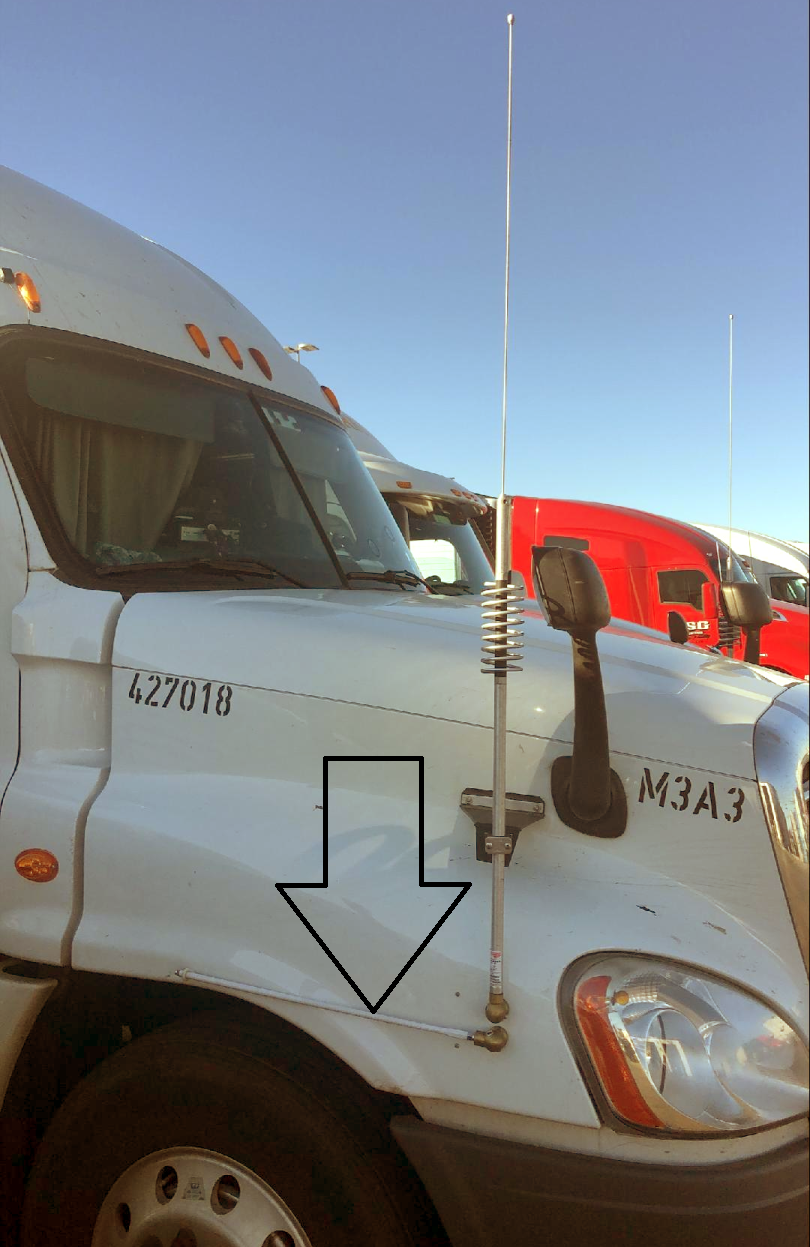James Rellinger  K5JPR  19Delta Transport, LLC  Dallas, TX

(Go Back)
============================================================================

Dick Reid, KK4OBI at QSL.net